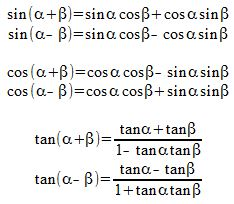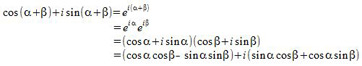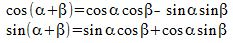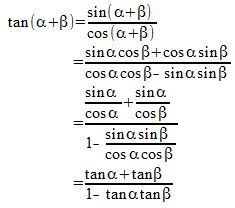# Proving the Addition & Subtraction Formulas for Sine, Cosine & Tangent

An error occurred trying to load this video.

Try refreshing the page, or contact customer support.

Coming up next: List of the Basic Trig Identities

### You're on a roll. Keep up the good work!

Replay
Your next lesson will play in 10 seconds
• 0:01 Addition & Subtraction…
• 1:28 Proof
• 3:33 Uses
• 4:27 Example
• 5:35 Lesson Summary

Want to watch this again later?

Timeline
Autoplay
Autoplay
Speed

#### Recommended Lessons and Courses for You

Lesson Transcript
Instructor: Yuanxin (Amy) Yang Alcocer

Amy has a master's degree in secondary education and has taught math at a public charter high school.

Watch this video lesson, and you will see how the angle sum and difference identities are derived for sine, cosine, and tangent. You will also learn how you can use these identities to help you solve problems.

## Addition & Subtraction Formulas

Trigonometry is full of identities or definitions. The ones that you are learning about right now have been proved over time and shown to be true. Because these identities have been mathematically derived with formal proofs, you can use these identities without worrying that they may give you a wrong answer.

The set of identities that we will look at today is called the angle sum and difference identities. These identities define how to turn a trig function of two angles added or subtracted from each other into a trig function of single angles. There are a total of six of these identities. Two for each of our trigonometric functions of sine, cosine, and tangent. There are two because we have one for addition and one for subtraction. Let's take a look at what they look like.The alpha and beta symbols represent angles written in either radians or degrees. Do your best to memorize these. It will help you in the long run on tests and exams. The key to memorizing these is to look for patterns.

For example, the cosine sum and difference identities splits into a pair of cosines and then a pair of sines. If we have the two angles added together, then our pair of sines will be subtracted from our pair of cosines. So, the sign flips for cosine. Looking at our sine identities we see that the sign stays. If we have the two angles being added, then the definition part also has a plus. What other patterns do you see?

## Proof

Remember how we talked about how these identities have been proven or derived mathematically so you can use these with confidence? Well, here is an overview of a simple way to prove these angle sum and difference identities. I don't expect you to fully understand these proofs as these derivations take you into the realm of higher math such as complex exponentials and imaginary numbers. But, if you can roughly see how these identities have been proven, then it will give you that much more assurance that these identities will work for you and will not fail you.

Let's take a look at the proof. It only requires four lines. It makes use of complex exponentials. It also makes use of the Euler formula, which states that e^ix = cos x + i sin x. Don't worry if these don't make sense now. Once you delve into higher math, such as calculus and higher, then you will understand how this all ties in with what you are learning now. Okay, so here is the proof:Study this proof carefully and we can see that yes, it uses the higher math skills, but it also uses our basic algebra skills to multiply things out. You might be asking how does this get to our identities? Well, take a look at the last line and see how we have two sets of parentheses. Now look at the very first line, the left side of the equation gives us our cosine sum and sine sum. If we set the cosine sum equal to the first set of parentheses in the last line, we see that we get our cosine sum identity. If we set the sine sum equal to the second set of parentheses in the fourth line, we see that we get the sine sum identity.To get our difference identities, we just replace our beta angle with a minus beta angle. To get our tangent identities, we make use of the definition of tangent in terms of sine and cosine. We know that tangent equals sine/cosine, so we simply write our tangent sum identity as the sine sum identity over the cosine sum identity. We then simplify to get to our tangent identity.Pretty neat, isn't it?

## Uses

Now you know that these identities are valid; what can you do with them? These identities are very useful for helping you to solve trig problems. Of course, with the help of calculators, you can solve any kind of problem by punching it in. But see, back in the day, people didn't have the use of handy graphing calculators that can do all kinds of fancy calculations. They had to work things out by hand. So, these identities helped them do that.

To unlock this lesson you must be a Study.com Member.

### Register to view this lesson

Are you a student or a teacher?

### Unlock Your Education

#### See for yourself why 30 million people use Study.com

##### Become a Study.com member and start learning now.
Back
What teachers are saying about Study.com

### Earning College Credit

Did you know… We have over 160 college courses that prepare you to earn credit by exam that is accepted by over 1,500 colleges and universities. You can test out of the first two years of college and save thousands off your degree. Anyone can earn credit-by-exam regardless of age or education level.